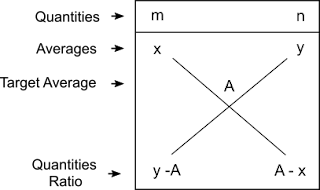# Alligation Rule and Mixtures and Replacements Formulas

Alligation Rule

Alligation rule helps us to find, in what ratio two mixtures with different concentrations are to be mixed to get a target concentration.

For example, we have two mixtures of alcohol concentrations with 40% and 85% and we need to find in what ratio these two are to be mixed to get 50% concentration.   To know this we have to learn about the weighted average.

Alligation Rule Derivation:
Let $m$ quantities have an average $x$ and $n$ quantities have an average $y$ are mixed together.To get the final Average $A$, the following weighted average formula to be used.
$$\displaystyle\frac{{{m} \times {x} + {n} \times {y}}}{{{m} + {n}}} = {A}$$
Let us re-arrange the terms above,
$\Rightarrow m \times x + n \times y = A(m + n)$
$\Rightarrow m \times x + n \times y = A \times m + A \times n$
$$\Rightarrow n \times y - A \times n = A \times m - m \times x$$
$$\Rightarrow n\left( {y - A} \right) = m\left( {A - x} \right)$$
$$\Rightarrow \dfrac{m}{n} = \dfrac{{y - A}}{{A - x}}$$
To easily apply the alligation rule, the following diagram is very useful.Here, $m,\,n$ are quantities or units to be taken, and $x$, $y$ are initial averages or concentrations, ($y > x$) and $A$ is the target average or concentration which lies in between $x$ and $y$.
Note: Alligation rule gives us only the ratio in which the initial mixtures are to be mixed to get the desired concentration but never gives the actual quantities.

Example 1
In what ratio milk with 40% concentration is mixed with another milk with concentration 60% to get 45% concentration?
Solution
Use alligation rule.Required ratio = $15 : 5$ = $3 : 1$

Example 2
How many liters of milk with 30% concentration is to be mixed with 20 liters of 70% concentration to get a mixture with 40% concentration?
Solution
Let the quantity of the first milk to be taken = $m$ liters.
Now, Use alligation rule.$\Rightarrow \dfrac{m}{{20}} = \dfrac{30}{10}$
$\Rightarrow m = 60$

Mixtures and Replacements

The problems related to mixtures based on two important concepts.  Alligation rule and Inverse proportionality rule are the two.

In these problems, we are asked to find the resultant concentration after mixing two or three components or the final concentration when one component of the mixture is being replaced by another component which is mostly one the components of the mixture.

Replacement Formula:
General formula for replacements is as follows: $FC = IC \times {\left( {1 - \dfrac{x}{V}} \right)^n}$
Here,
FC = Final concentration
IC = Initital concentration
x = replacement quantity
V = Final volume after replacement
n = number of replacements

Note:  Always remember FC and IC are the concentrations of the second component in the mixture.  "x" is the concentration of the first component.